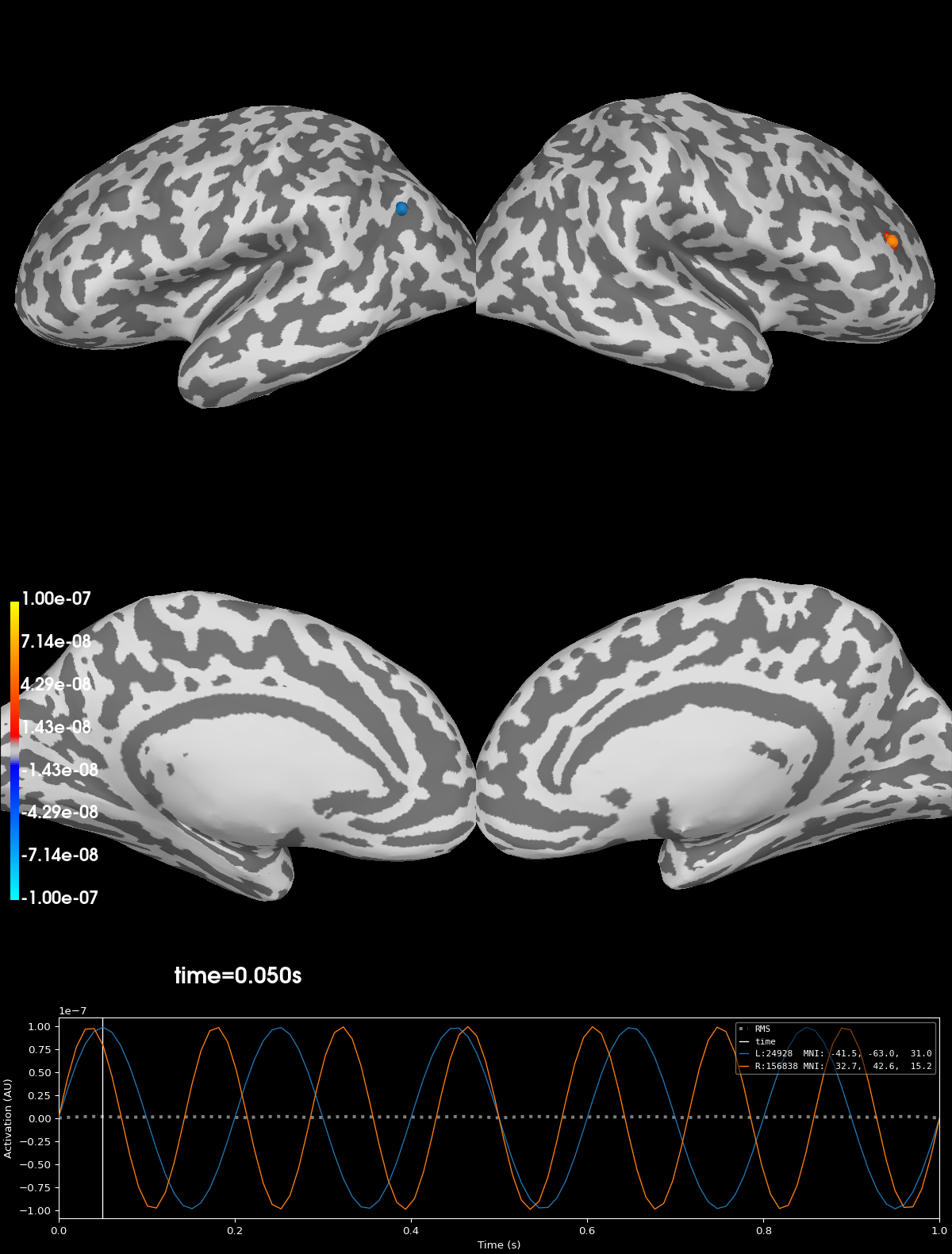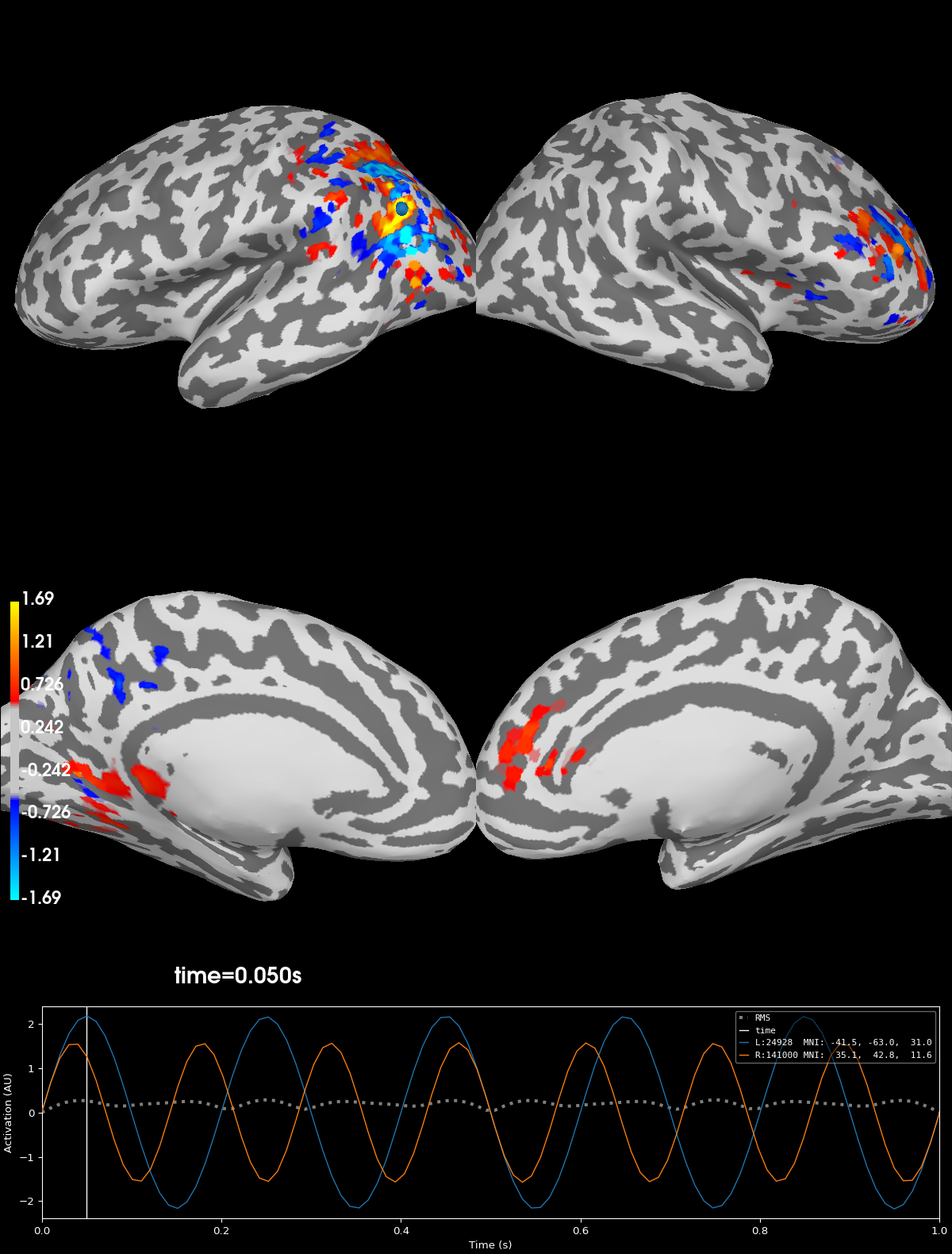# Corrupt known signal with point spread#

The aim of this tutorial is to demonstrate how to put a known signal at a desired location(s) in a `mne.SourceEstimate` and then corrupt the signal with point-spread by applying a forward and inverse solution.

```import numpy as np

import mne
from mne.datasets import sample

from mne.simulation import simulate_stc, simulate_evoked
```

First, we set some parameters.

```seed = 42

# parameters for inverse method
method = "sLORETA"
snr = 3.0
lambda2 = 1.0 / snr**2

# signal simulation parameters
# do not add extra noise to the known signals
nave = np.inf
T = 100
times = np.linspace(0, 1, T)
dt = times - times

# Paths to MEG data
data_path = sample.data_path()
subjects_dir = data_path / "subjects"
fname_fwd = data_path / "MEG" / "sample" / "sample_audvis-meg-oct-6-fwd.fif"
fname_inv = data_path / "MEG" / "sample" / "sample_audvis-meg-oct-6-meg-fixed-inv.fif"

fname_evoked = data_path / "MEG" / "sample" / "sample_audvis-ave.fif"
```

```fwd = mne.read_forward_solution(fname_fwd)
fwd = mne.convert_forward_solution(fwd, force_fixed=True, surf_ori=True, use_cps=False)

raw = mne.io.read_raw_fif(data_path / "MEG" / "sample" / "sample_audvis_raw.fif")

raw.set_eeg_reference(projection=True)
events = mne.find_events(raw)
event_id = {"Auditory/Left": 1, "Auditory/Right": 2}
epochs = mne.Epochs(raw, events, event_id, baseline=(None, 0), preload=True)
evoked = epochs.average()

label_names = [label.name for label in labels]
n_labels = len(labels)
```
```Reading forward solution from /home/circleci/mne_data/MNE-sample-data/MEG/sample/sample_audvis-meg-oct-6-fwd.fif...
Computing patch statistics...
[done]
Computing patch statistics...
[done]
Desired named matrix (kind = 3523) not available
Read MEG forward solution (7498 sources, 306 channels, free orientations)
Source spaces transformed to the forward solution coordinate frame
Changing to fixed-orientation forward solution with surface-based source orientations...
[done]
Reading inverse operator decomposition from /home/circleci/mne_data/MNE-sample-data/MEG/sample/sample_audvis-meg-oct-6-meg-fixed-inv.fif...
[done]
[done]
305 x 305 full covariance (kind = 1) found.
Read a total of 4 projection items:
PCA-v1 (1 x 102) active
PCA-v2 (1 x 102) active
PCA-v3 (1 x 102) active
Average EEG reference (1 x 60) active
7498 x 7498 diagonal covariance (kind = 2) found.
Did not find the desired covariance matrix (kind = 6)
7498 x 7498 diagonal covariance (kind = 5) found.
Did not find the desired covariance matrix (kind = 3)
Computing patch statistics...
[done]
Computing patch statistics...
[done]
Read a total of 4 projection items:
PCA-v1 (1 x 102) active
PCA-v2 (1 x 102) active
PCA-v3 (1 x 102) active
Average EEG reference (1 x 60) active
Source spaces transformed to the inverse solution coordinate frame
Opening raw data file /home/circleci/mne_data/MNE-sample-data/MEG/sample/sample_audvis_raw.fif...
Read a total of 3 projection items:
PCA-v1 (1 x 102)  idle
PCA-v2 (1 x 102)  idle
PCA-v3 (1 x 102)  idle
Range : 25800 ... 192599 =     42.956 ...   320.670 secs
EEG channel type selected for re-referencing
1 projection items deactivated
320 events found
Event IDs: [ 1  2  3  4  5 32]
145 matching events found
Setting baseline interval to [-0.19979521315838786, 0.0] s
Applying baseline correction (mode: mean)
Created an SSP operator (subspace dimension = 4)
4 projection items activated
NOTE: pick_channels() is a legacy function. New code should use inst.pick(...).
```

## Estimate the background noise covariance from the baseline period#

```cov = mne.compute_covariance(epochs, tmin=None, tmax=0.0)
```
```Computing rank from data with rank=None
Using tolerance 1.4e-08 (2.2e-16 eps * 306 dim * 2.1e+05  max singular value)
Estimated rank (mag + grad): 303
MEG: rank 303 computed from 306 data channels with 3 projectors
Using tolerance 4.9e-11 (2.2e-16 eps * 60 dim * 3.7e+03  max singular value)
Estimated rank (eeg): 59
EEG: rank 59 computed from 60 data channels with 1 projector
Created an SSP operator (subspace dimension = 4)
Setting small MEG eigenvalues to zero (without PCA)
Setting small EEG eigenvalues to zero (without PCA)
Reducing data rank from 366 -> 362
Estimating covariance using EMPIRICAL
Done.
Number of samples used : 17545
[done]
```

## Generate sinusoids in two spatially distant labels#

```# The known signal is all zero-s off of the two labels of interest
signal = np.zeros((n_labels, T))
idx = label_names.index("inferiorparietal-lh")
signal[idx, :] = 1e-7 * np.sin(5 * 2 * np.pi * times)
idx = label_names.index("rostralmiddlefrontal-rh")
signal[idx, :] = 1e-7 * np.sin(7 * 2 * np.pi * times)
```

## Find the center vertices in source space of each label#

We want the known signal in each label to only be active at the center. We create a mask for each label that is 1 at the center vertex and 0 at all other vertices in the label. This mask is then used when simulating source-space data.

```hemi_to_ind = {"lh": 0, "rh": 1}
for i, label in enumerate(labels):
# The `center_of_mass` function needs labels to have values.
labels[i].values.fill(1.0)

# Restrict the eligible vertices to be those on the surface under
# consideration and within the label.
surf_vertices = fwd["src"][hemi_to_ind[label.hemi]]["vertno"]
restrict_verts = np.intersect1d(surf_vertices, label.vertices)
com = labels[i].center_of_mass(
subjects_dir=subjects_dir, restrict_vertices=restrict_verts, surf="white"
)

# Convert the center of vertex index from surface vertex list to Label's
# vertex list.
cent_idx = np.where(label.vertices == com)

# Create a mask with 1 at center vertex and zeros elsewhere.
labels[i].values.fill(0.0)
labels[i].values[cent_idx] = 1.0

if "transversetemporal" in label.name:
dist, _ = label.distances_to_outside(subjects_dir=subjects_dir)
dist = dist[cent_idx]
area = label.compute_area(subjects_dir=subjects_dir)
# convert to equivalent circular radius
r = np.sqrt(area / np.pi)
print(
f"{label.name} COM vertex is {dist * 1e3:0.1f} mm from edge "
f"(label area equivalent to a circle with r={r * 1e3:0.1f} mm)"
)
```
```    Triangle neighbors and vertex normals...
transversetemporal-lh COM vertex is 7.5 mm from edge (label area equivalent to a circle with r=11.5 mm)
Triangle neighbors and vertex normals...
transversetemporal-rh COM vertex is 6.8 mm from edge (label area equivalent to a circle with r=10.8 mm)
```

## Create source-space data with known signals#

Put known signals onto surface vertices using the array of signals and the label masks (stored in labels[i].values).

```stc_gen = simulate_stc(fwd["src"], labels, signal, times, dt, value_fun=lambda x: x)
```

## Plot original signals#

Note that the original signals are highly concentrated (point) sources.

```kwargs = dict(
subjects_dir=subjects_dir,
hemi="split",
smoothing_steps=4,
time_unit="s",
initial_time=0.05,
size=1200,
views=["lat", "med"],
)
clim = dict(kind="value", pos_lims=[1e-9, 1e-8, 1e-7])
brain_gen = stc_gen.plot(clim=clim, **kwargs)
```## Simulate sensor-space signals#

Use the forward solution and add Gaussian noise to simulate sensor-space (evoked) data from the known source-space signals. The amount of noise is controlled by `nave` (higher values imply less noise).

```evoked_gen = simulate_evoked(fwd, stc_gen, evoked.info, cov, nave, random_state=seed)

# Map the simulated sensor-space data to source-space using the inverse
# operator.
stc_inv = apply_inverse(evoked_gen, inv_op, lambda2, method=method)
```
```Projecting source estimate to sensor space...
[done]
4 projection items deactivated
Created an SSP operator (subspace dimension = 3)
4 projection items activated
SSP projectors applied...
Preparing the inverse operator for use...
Scaled noise and source covariance from nave = 1 to nave = 1
Created the regularized inverter
Created an SSP operator (subspace dimension = 3)
Created the whitener using a noise covariance matrix with rank 302 (3 small eigenvalues omitted)
Computing noise-normalization factors (sLORETA)...
[done]
Applying inverse operator to ""...
Picked 305 channels from the data
Computing inverse...
Eigenleads need to be weighted ...
Computing residual...
Explained  99.7% variance
sLORETA...
[done]
```

## Plot the point-spread of corrupted signal#

Notice that after applying the forward- and inverse-operators to the known point sources that the point sources have spread across the source-space. This spread is due to the minimum norm solution so that the signal leaks to nearby vertices with similar orientations so that signal ends up crossing the sulci and gyri.

```brain_inv = stc_inv.plot(**kwargs)
``````Using control points [0.45968308 0.57021267 1.69354621]
```

## Exercises#

• Change the `method` parameter to either `'dSPM'` or `'MNE'` to explore the effect of the inverse method.

• Try setting `evoked_snr` to a small, finite value, e.g. 3., to see the effect of noise.

Total running time of the script: ( 0 minutes 23.657 seconds)

Estimated memory usage: 452 MB

Gallery generated by Sphinx-Gallery